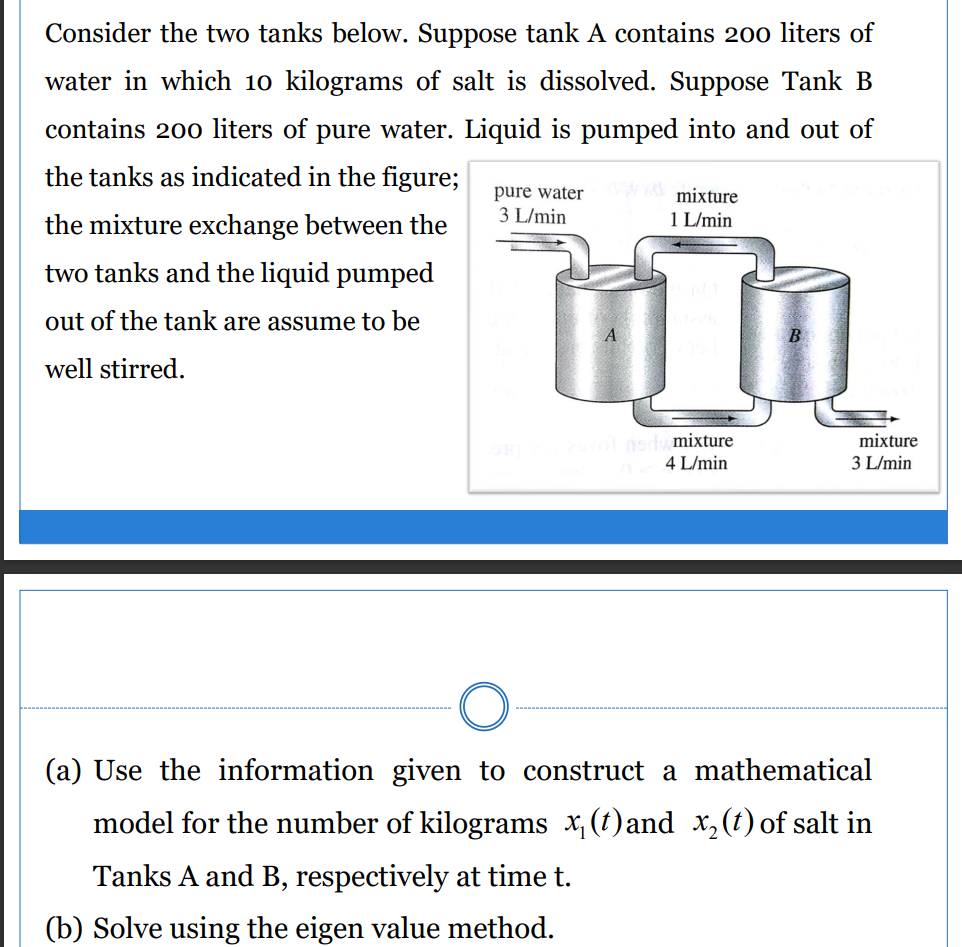### Create an Account

Already have account?

### Forgot Your Password ?

Home / Questions / Consider the two tanks below. Suppose tank A contains 200 liters of water in which 10 kilo...

# Consider the two tanks below. Suppose tank A contains 200 liters of water in which 10 kilograms of salt is dissolved. Suppose Tank B contains 200 liters of pure water. Liquid is pumped into and out of

Consider the two tanks below. Suppose tank A contains 200 liters of water in which 10 kilograms of salt is dissolved. Suppose Tank B contains 200 liters of pure water. Liquid is pumped into and out of the tanks as indicated in the figure; mixture 3 L/min the mixture exchange between the two tanks and the liquid pumped out of the tank are assume to be pure water 1 L/min well stirred. Smixture 4 L/min mixture 3 L/min (a) Use the information given to construct a mathematical model for the number of kilograms x,(t)and xz(t) of salt in Tanks A and B, respectively at time t. (b) Solve using the eigen value method.Apr 15 2021 View more View Less

#### Answer (Solved)Subscribe To Get Solution